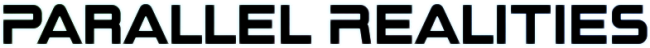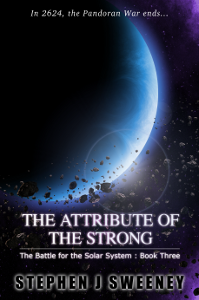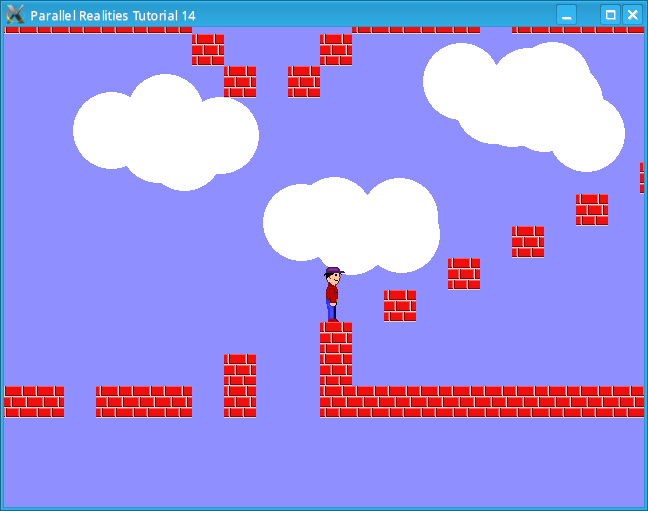Android Games Latest Updates SDL2 Santa game tutorial 🎅 Thu, 23rd November 2023 SDL2 Shooter 3 tutorial Wed, 15th February 2023 The Legend of Edgar 1.36 Sun, 1st January 2023 SDL2 map editor tutorial [UPDATED] Sat, 10th September 2022 TBFTSS: The Pandoran War - Amiga OS4 Port Wed, 7th September 2022 All Updates » Tags • android (3)• battle-for-the-solar-system (10)• blob-wars (10)• brexit (1)• code (6)• edgar (8)• games (40)• lasagne-monsters (1)• making-of (5)• match3 (1)• numberblocksonline (1)• orb (2)• site (1)• tanx (4)• three-guys (3)• three-guys-apocalypse (3)• tutorials (15)• water-closet (4) BooksThe Attribute of the Strong (Battle for the Solar System, #3) The Pandoran War is nearing its end... and the Senate's Mistake have all but won. Leaving a galaxy in ruin behind them, they set their sights on Sol and prepare to finish their twelve year Mission. All seems lost. But in the final forty-eight hours, while hunting for the elusive Zackaria, the White Knights make a discovery in the former Mitikas Empire that could herald one last chance at victory. Intermediate Tutorials Intermediate Game Tutorial #4 - Tile based map collision detectionIntroduction This tutorial deals with collision detection. Compile and run tutorial14. The program will read the map data file and display the map on the screen. Use the arrow keys (not the ones on the numeric pad) to move the character around the screen. Pressing space will make the character jump. Should the character fall out of the map screen it will reappear after a couple of seconds. An in-depth look We reintroduce the Entity structure and add a few extra variables to it: ```typedef struct Entity { int w, h, onGround; int thinkTime; float x, y, dirX, dirY; } Entity; ``` The onGround variable is used to determine if the Entity is on the ground. We use this to determine whether or not the Entity is allowed to jump or perform any other ground based action. dirX and dirY are used to apply directional force to the x and y variables. This is important when working with gravity or friction. We will see how this is used later. We will only look at three files in this tutorial since the other files and functions have been covered numerous times in previous tutorials. map.c contains an extra function to center an Entity on the screen: ```void centerEntityOnMap(Entity *e) { map.startX = e->x - (SCREEN_WIDTH / 2); if (map.startX < 0) { map.startX = 0; } else if (map.startX + SCREEN_WIDTH >= map.maxX) { map.startX = map.maxX - SCREEN_WIDTH; } map.startY = e->y - (SCREEN_HEIGHT / 2); if (map.startY < 0) { map.startY = 0; } else if (map.startY + SCREEN_HEIGHT >= map.maxY) { map.startY = map.maxY - SCREEN_HEIGHT; } } ``` We take the Entity's current horizontal position, subtract half the screen's width from it and assign this to startX. We then perform the usual bounds check to make sure that the startX value to make sure we don't attempt to draw non existant parts of the map. We do the same with the vertical position. We will now look at the code to handle the player, which is in player.c: ```void doPlayer() { if (player.thinkTime == 0) { player.dirX = 0; /* Gravity always pulls the player down */ player.dirY += GRAVITY_SPEED; if (player.dirY >= MAX_FALL_SPEED) { player.dirY = MAX_FALL_SPEED; } if (input.left == 1) { player.dirX -= PLAYER_SPEED; } else if (input.right == 1) { player.dirX += PLAYER_SPEED; } if (input.jump == 1) { if (player.onGround == 1) { player.dirY = -11; } input.jump = 0; } checkToMap(&player); centerEntityOnMap(&player); } if (player.thinkTime > 0) { player.thinkTime--; if (player.thinkTime == 0) { initPlayer(); } } } ``` The first check we perform is that the player's thinkTime is greater than 0 and if it is then we can perform actions on it. We first set the dirX to 0. This means that the player will stop moving instantly when we release the key. If we wanted to make the player slowly stop then we could do something like the following: ```player.dirX *= 0.98; ``` This would mean that when we released the arrow key the player would take a few frames to come to a complete halt. This could be used to give the illusion that the player is on ice where there is a low coefficient of friction. Next we apply gravity to the player's vertical movement. Not that we do not simply set the dirY to the amount of gravitational pull, but we increment it instead. This will mean that anything currently moving up will start to be pulled down after a while. We also limit the maximum speed at which the player will fall. The left and right movements should be self explanitory. When jump is detected we need to first check if the player is on the ground, otherwise they could jump any time they wanted, which is undesirable. Provided they can jump, we set the dirY to -11, which in this game is a reasonable amount to jump by. Note that this value will be decremented by gravity in the following frames. Finally, we call checkToMap and centerEntityOnMap. We will look at checkToMap shortly. If the player's thinkTime is greater than 0 then the player cannot perform any actions and we simply decrease the value. Once it hits 0 we call initPlayer to reset the player on the map. We will now look at the map collision detection. This function is stored in collisions.c: ```void checkToMap(Entity *e) { int i, x1, x2, y1, y2; /* Remove the user from the ground */ e->onGround = 0; /* Test the horizontal movement first */ i = e->h > TILE_SIZE ? TILE_SIZE : e->h; for (;;) { x1 = (e->x + e->dirX) / TILE_SIZE; x2 = (e->x + e->dirX + e->w - 1) / TILE_SIZE; y1 = (e->y) / TILE_SIZE; y2 = (e->y + i - 1) / TILE_SIZE; if (x1 >= 0 && x2 < MAX_MAP_X && y1 >= 0 && y2 < MAX_MAP_Y) { if (e->dirX > 0) { /* Trying to move right */ if ((map.tile[y1][x2] != BLANK_TILE) || (map.tile[y2][x2]!=BLANK_TILE)) { /* Place the player as close to the solid tile as possible */ e->x = x2 * TILE_SIZE; e->x -= e->w + 1; e->dirX = 0; } } else if (e->dirX < 0) { /* Trying to move left */ if ((map.tile[y1][x1] != BLANK_TILE) || (map.tile[y2][x1]!=BLANK_TILE)) { /* Place the player as close to the solid tile as possible */ e->x = (x1 + 1) * TILE_SIZE; e->dirX = 0; } } } if (i == e->h) { break; } i += TILE_SIZE; if (i > e->h) { i = e->h; } } /* Now test the vertical movement */ i = e->w > TILE_SIZE ? TILE_SIZE : e->w; for (;;) { x1 = (e->x) / TILE_SIZE; x2 = (e->x + i) / TILE_SIZE; y1 = (e->y + e->dirY) / TILE_SIZE; y2 = (e->y + e->dirY + e->h) / TILE_SIZE; if (x1 >= 0 && x2 < MAX_MAP_X && y1 >= 0 && y2 < MAX_MAP_Y) { if (e->dirY > 0) { /* Trying to move down */ if ((map.tile[y2][x1] != BLANK_TILE) || (map.tile[y2][x2]!=BLANK_TILE)) { /* Place the player as close to the solid tile as possible */ e->y = y2 * TILE_SIZE; e->y -= e->h; e->dirY = 0; e->onGround = 1; } } else if (e->dirY < 0) { /* Trying to move up */ if ((map.tile[y1][x1] != BLANK_TILE) || (map.tile[y1][x2]!=BLANK_TILE)) { /* Place the player as close to the solid tile as possible */ e->y = (y1 + 1) * TILE_SIZE; e->dirY = 0; } } } if (i == e->w) { break; } i += TILE_SIZE; if (i > e->w) { i = e->w; } } /* Now apply the movement */ e->x += e->dirX; e->y += e->dirY; if (e->x < 0) { e->x = 0; } else if (e->x + e->w >= map.maxX) { e->x = map.maxX - e->w - 1; } if (e->y > map.maxY) { e->thinkTime = 60; } } ``` This function is fairly complex, so we will look at it in sections. Firstly, we set the onGround variable to 0. During our map tests we may set this variable back to 1. The easiest way to test map collisions is to check the horizontal and vertical movements separately. We will start with the horizontal movement. Determining whether or not an Entity has collided with a map block is a case of checking the value of the map tile that each of the 4 corners of the Entity will be in after the movement has taken place. This approach will only work though if the Entity's horizontal and vertical sizes are less than or equal to the TILE_SIZE, otherwise a very large Entity will be able to move through the map tiles since its corners may not necessarily collide with a tile even though its midsection does. To get around this problem we will break the Entity's height into portions: ```i = e->h > TILE_SIZE ? TILE_SIZE : e->h; ``` In our example, the player sprite is 55 pixels tall, i will initially be TILE_SIZE. We next enter a loop and calculate the corners that the player will be in after it has moved: ```x1 = (e->x + e->dirX) / TILE_SIZE; x2 = (e->x + e->dirX + e->w - 1) / TILE_SIZE; y1 = (e->y) / TILE_SIZE; y2 = (e->y + i - 1) / TILE_SIZE; ``` x1 is the left side, x2 is the right side, y1 is the top side and y2 is the bottom. We will combine these values together to calculate the coordinates of the corners. Next we check that the values the 4 sides are within the bounds of the tile array. If they are not then we skip over the calculation since the player has gone outside the bounds of the screen, either because they have jumped at the top of the screen or they may have fallen out of the map, and we don't want to attempt to index an array outside of its bounds. Provided the values are legal we then check if the player is moving right: ```if (e->dirX > 0) { /* Trying to move right */ if ((map.tile[y1][x2] != BLANK_TILE) || (map.tile[y2][x2] != BLANK_TILE)) { /* Place the player as close to the solid tile as possible */ e->x = x2 * TILE_SIZE; e->x -= e->w + 1; e->dirX = 0; } } ``` We want to check the top right and bottom right corners of the Entity so we use y1 and y2 for the top and bottom values and x2 since it is the right side of the Entity. We then check the tile type at these two corners and if either of the tiles is not empty, then we have hit a map tile. We then move the Entity as close to the tile as possible and set the dirX to 0. If we are moving left then the code is fairly similar: ```else if (e->dirX < 0) { /* Trying to move left */ if ((map.tile[y1][x1] != BLANK_TILE) || (map.tile[y2][x1] != BLANK_TILE)) { /* Place the player as close to the solid tile as possible */ e->x = (x1 + 1) * TILE_SIZE; e->dirX = 0; } } ``` We still use y1 and y2 but we use x1 since this is the left side of the Entity. Next we check if we have to test the next block of the body. ```/* Exit this loop if we have tested all of the body */ if (i == e->h) { break; } /* Test the next block */ i += TILE_SIZE; if (i > e->h) { i = e->h; } ``` If the current value of i is equal to the Entity's height then we have completed testing the horizontal movement and can exit the loop. Otherwise we increment i by TILE_SIZE to test the next block. If i is greater than the Entity's height then we set it to the Entity's height since we don't want to test outside of this. We then test the vertical movement, which is similar to the horizontal checking, except that during the vertical checking, if we are moving down and we encounter a solid tile, we set the onGround variable to 1. Finally, we apply the dirX and dirY to allow the Entity to move: ```/* Now apply the movement */ e->x += e->dirX; e->y += e->dirY; if (e->x < 0) { e->x = 0; } else if (e->x + e->w >= map.maxX) { e->x = map.maxX - e->w - 1; } if (e->y > map.maxY) { e->thinkTime = 60; } ``` Note that dirX and dirY may have been set to 0 in which case no movement will take place. We also prevent the player from being able to move off the left and right hand edges of the map. If the player's y variable is greater than the maxY of the map then the Entity has fallen out of the map, in which case we set the Entity's thinkTime to 60, which is about 1 second. As seen earlier, this will make the player reappear at the start of the map. Conclusion The code for checking the map may seem very long, but in reality we are simply performing the same check 4 times, one for each corner. You can use the map editor in the previous tutorial to modify the map included in this tutorial. In the following tutorials we will look at more animation and additions to the map. Downloads Copyright © Parallel Realities, 2001 - 2023. All Rights Reserved. Last Updated: 23rd November 2023